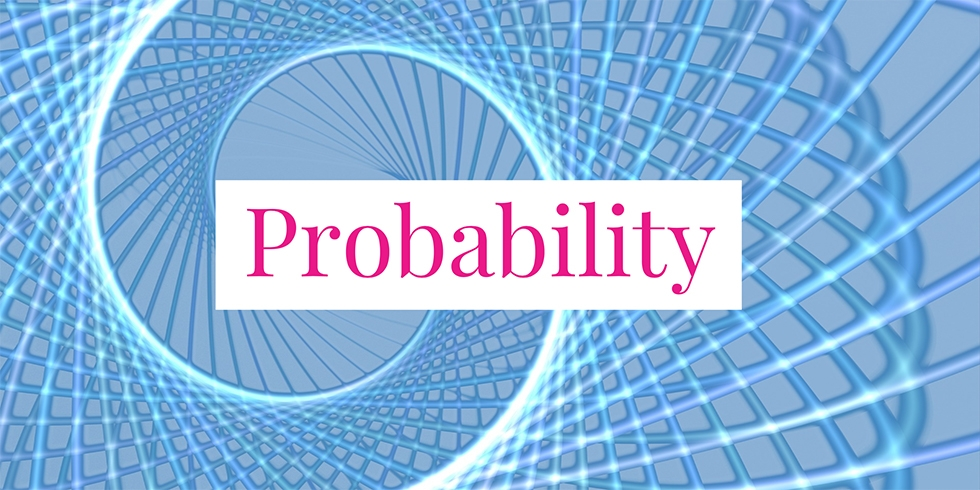# Conditional Probability Problems about Die Rolling## Problem 728

A fair six-sided die is rolled.

(1) What is the conditional probability that the die lands on a prime number given the die lands on an odd number?

(2) What is the conditional probability that the die lands on 1 given the die lands on a prime number?Add to solve later

## Solution.

Let $E$ and $F$ be events. Then the conditional probability that $E$ occurs given $F$ has occurred, which is denoted by $P(E\mid F)$, is defined as
$P(E \mid F) = \frac{P(E \cap F)}{P(F)}.$

For uniform space (such as fair die rolling), this can be rewritten as
$P(E \mid F) = \frac{|E \cap F|}{|F|}.$ Here the notation $|A|$ means the number of elements in $A$.

### Solution (1)

Let $E = \{2, 3, 5\}$ be the set of prime number faces of a die. Let $F = \{1, 3, 5\}$ be the set of odd faces of a die. Then the required conditional probability can be calculated as follows:
\begin{align*}
P(E \mid F) &= \frac{|E \cap F|}{|F|}\\
&= \frac{|\{2, 3, 5\} \cap \{1, 3, 5\}|}{|\{1, 3, 5\}|}\\
&= \frac{|\{3, 5\}|}{\{1,3,5\}|}\\
&= \frac{2}{3}
\end{align*}

### Solution (2)

The required conditional probability is
\begin{align*}
P(\{1\} \mid E) &= \frac{|\{1\} \cap E|}{|E|}\\
&= \frac{|\{1\} \cap \{2, 3, 5\}|}{|\{2, 3, 5\}|}\\
&= \frac{|\emptyset|}{|\{2, 3, 5\}|}\\
&= \frac{0}{3} = 0
\end{align*}Add to solve later

### More from my site

#### You may also like...

This site uses Akismet to reduce spam. Learn how your comment data is processed.

###### More in Probability##### Probability Problems about Two Dice

Two fair and distinguishable six-sided dice are rolled. (1) What is the probability that the sum of the upturned faces...

Close